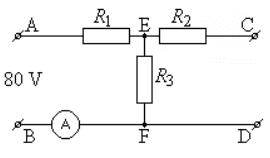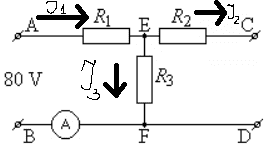# Finding the current

## Homework Statement

There is a circuit which is shown in the picture. ##R_1=10 Ω;## ##R_2=10 Ω;## ##R_3=30 Ω##.
I need to find what ampermeter is showing.U=IR

## The Attempt at a SolutionI am trying to use kirchhoff rules here.

##I_1=I_2+I_3##
##I_1R_1+I_3R_3=80##
##I_2R_2=I_3R_3##

I get that ##I_3=1.14A##. Is this correct?

Assuming C & D are open as shown, I2 should be 0.

•CWatters
But how do I know whether there is voltage between C and D? It is not stated in the problem. In fact, the next question of this problem asks to find the voltage between C & D.

NascentOxygen
Staff Emeritus
There will be a voltage between C and D, even though I2 = 0.

CWatters
Homework Helper
Gold Member
I am trying to use kirchhoff rules here.
snip
I2R2=I3R3

That last line isn't correct. The voltage across R2 and R3 are not the same.

There is nothing connected to C and D so no current flows into or out of C and D. So I2 = 0. Think what that means for I1 and I3.